# Say Hello to the User

Writing a Python script that responds to a user is as easy as using the input() function
Project Example: Show Me Nearest Earthquakes
A step-by-step process of creating a project that downloads earthquake data, geocodes a user's location, and displays the nearest locations.

# Getting started

Change into your compciv-2016/projects/show-me-where directory (run the `pwd` command to double-check).

```    compciv-2016
└── projects
└── show-me-where
```

Using Sublime Text, or whatever you're using to create new text files, create a file named: `woz.py`:

```    compciv-2016
└── projects
└── show-me-where
└── woz.py
```

# The input() function

The built-in input() function is used to capture user input. Put this in `woz.py`:

``````usertext = input()
print("Hello", usertext)
``````

Switch to your system shell and run:

``````\$ python woz.py
``````

Then run `python woz.py`; you should see this kind of interaction: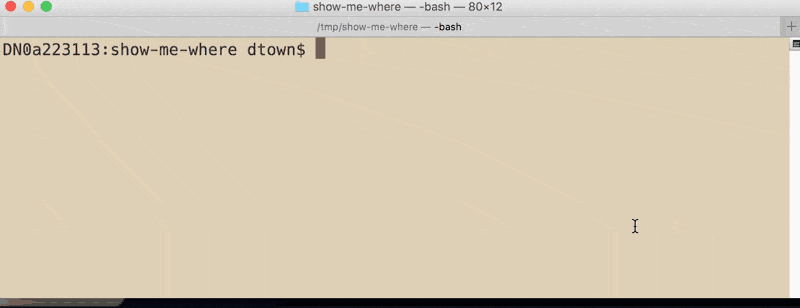Congrats, you've made a program that interacts with a user. It doesn't get much more complicated than that (unless you want it to).

The `input()` function takes an optional argument: a text string which can be used to prompt the user, e.g. `"Hey whats ur name?"`. This results in a slightly more user-friendly interface. Modify `woz.py` to your taste:

``````usertext = input("What is your name?")
print("Hello", usertext)
``````

Rerunning `woz.py` will get you this interaction: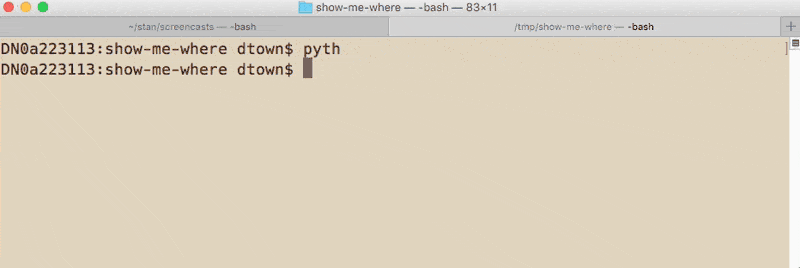It's kind of annoying how the prompt butts up against the user-supplied value:

``````What is your name?Donald
``````

We can fix that by adding a space after the prompt, i.e. after the `?`:

``````usertext = input("What is your name? ")
print("Hello", usertext)
``````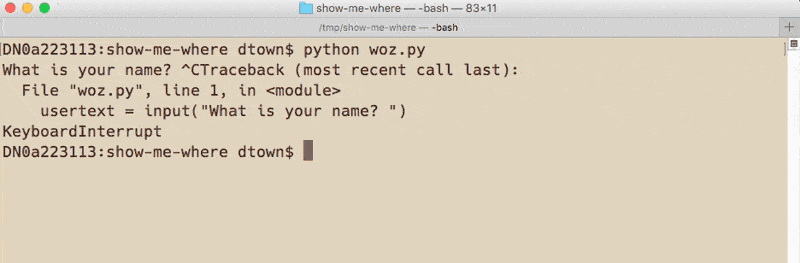And we can sass up the output. For example, to make the Terminal display bold text, we prepend and append a couple of specific text strings to what we want to make bold:

``````B_X = "\033[1m"
B_Z = "\033[0m"
usertext = input("What is your name? ")
print("Hello", B_X + usertext + B_Z)
``````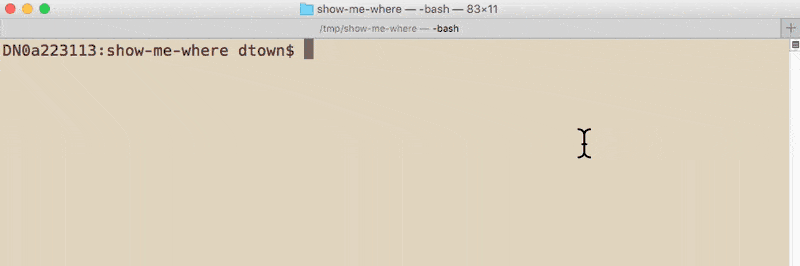So our program knows how to say "hello" to a user, but we want it to do more than just that. For example, the program should ask the user what the user wants to do.

If the user responds with `"hello"`, do what we've done above.

If the user responds with anything else…for now, let's just tell them we don't know how to respond to that.

This is nothing more than using an if- and else-statement, and using two `input()` calls:

1. To ask the user what to do.
2. If the user wants to say `"hello"`, then ask the user for their name (as before).

Here's one way to do it:

``````B_X = "\033[1m"
B_Z = "\033[0m"

the_command = input("What do you want to do? ")
if the_command == "hello":
usertext = input("What is your name? ")
print("Hello", B_X + usertext + B_Z)
else:
print("Sorry, I don't know how to respond to", the_command)

``````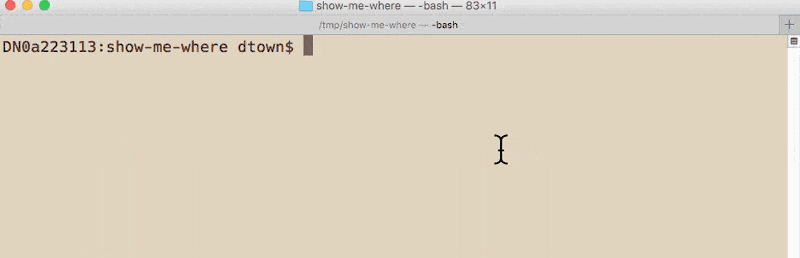# Filling out placeholder branches

Our program is required to respond to several commands. We haven't written the logic for any of those commands, but we can still write the `if/elif/else` logic.

In the next step, we'll write out the branch for the `"geocode"` option, so at a minimum, we can add that before moving on:

``````B_X = "\033[1m"
B_Z = "\033[0m"

the_command = input("What do you want to do? ")
if the_command == "hello":
usertext = input("What is your name? ")
print("Hello", B_X + usertext + B_Z)

elif the_command == 'geocode':
print("TODO: geocode it")

else:
print("Sorry, I don't know how to respond to", the_command)

``````

Running woz.py and trying out `"geocode"`:

``````\$ python woz.py
What do you want to do? geocode
TODO: geocode it
``````

That's good enough for now.

# Moving on

Our program doesn't do much, but we've built the framework for making something that can now do some neat things for the user, just by using a some `if/elif/else` branch logic.